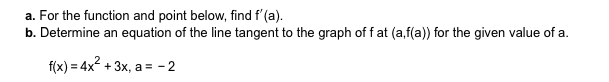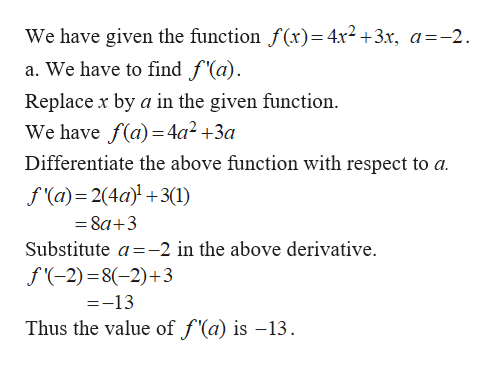# a. For the function and point below, find f'(a)b. Determine an equation of the line tangent to the graph of f at (a,f(a)) for the given value of af(x) 4x2+3x, a-2

Question
3 viewshelp_outlineImage Transcriptionclosea. For the function and point below, find f'(a) b. Determine an equation of the line tangent to the graph of f at (a,f(a)) for the given value of a f(x) 4x2+3x, a-2 fullscreen
check_circle

star
star
star
star
star
1 Rating
Step 1help_outlineImage TranscriptioncloseWe have given the function f(x)= 4x2 +3x, a=-2 a. We have to find f'(a) Replacex by a in the given function We have f(a) 4a2 +3a Differentiate the above function with respect to a f (a) 2(4a)3(1) 8a+3 Substitute a-2 in the above derivative f-2) 8(-2)+3 =-13 Thus the value of f'(a) is -13 fullscreen

### Want to see the full answer?

See Solution

#### Want to see this answer and more?

Solutions are written by subject experts who are available 24/7. Questions are typically answered within 1 hour.*

See Solution
*Response times may vary by subject and question.
Tagged in

### Calculus